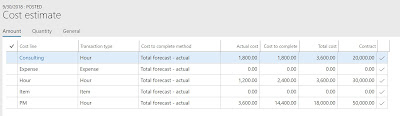# Calculation Method and Balancing Fee Journal

There are three calculation methods when it comes to fixed price projects, they are:

1. None
2. Markup percent total
3. Markup percent estimate line
This post is not about describing what each of the calculation method does but this post is the super summary of when does the system create the balancing fee journal and when it does not. This post is for the advanced users of revenue recognition functionality. It is assumed that the readers of this post are aware of all the three methods and how they behave. This is a small post to just present the gist in a table format with respect to the creation of balancing fee journal for all three calculation methods.Calculation method and Balancing fee journal
System behavior is different when the:
• dimensions are NOT present on the project and hours transactions and
• dimensions on the project and hours transactions are different
No dimensions on the project and hours transactions
Contract value – 100,000
Hours forecast – 146
No dimensions on the project
Contract value distributed during the estimateContract value distribution among cost lines
Cost posted for all the three cost lines are 1,800, 1,200 and 3,600, which is equal to 6,600. Based on the contract value distribution, the revenue recognized should be 30,000. [((1,800 / 3,600) *20,000) + ((1,200 / 3,600) *30,000)) + ((3,600 / 18,000) *50,000))]
System suggested percent complete
But system suggested percent complete is 27.40 [((Total posted hours / Total hours forecast in Qty)*100), which is (40/146)*100 = 27.40] hence the accrued revenue value would be 27,400. Hence the balancing fee journal should be approximately 2,600. Completion is based on ‘Quantity’ in the cost template.
When the estimate is posted, following is the voucher posted:
And the posted transactions are as below, which shows the actual 30,000 accrued revenue and the balancing fee transaction of approximately 2,600. However strange enough there is no voucher for that balancing transaction.
Dimensions on the project and hours transaction are different

I repeated the above example with the same values, but this time with the dimensions on the project and hours transaction. However the ‘Department’ dimension  on the project is 22, and on all the three hours transactions are 23, 24 and 25 respectively.
With all the steps same as above, when the estimate was created, the system showed a percent complete of 27.40. I manually changed to 25 to see the impact on the posting.Percent complete changed from automatic to manual
The voucher that was posted was expected to have a balancing fee transaction of 5000, with the dimensions from the project.
Posted transaction was as below:
However in this case the voucher was posted for the balancing fee transaction.

Hopefully this post has helped you get more insight into the revenue recognition and generation of balancing fee transaction.
Kind Regards
Sarang Kusare